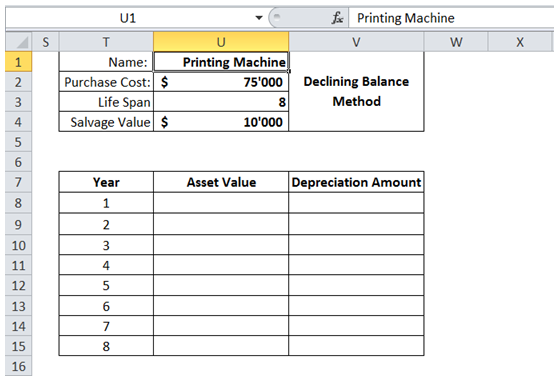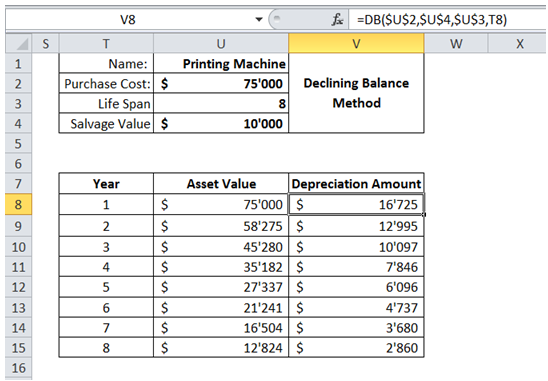## Calculate depreciation of assets in Excel

For example, I have a Printing Machine whose original cost is \$75'000, useful life is 8 years and Salvage cost is \$10'000. I would like to know the asset value and depreciation amount over its useful life using Declining Balance for calculations.To do it in Excel, here is the answer:

a) Enter the formula =DB(\$U\$2,\$U\$4,\$U\$3,T8) for Depreciation Amount of first year.

The first argument is the Purchase Cost, second corresponds to Salvage Value, 3rd corresponds to Life Span in years.

Fourth argument is the period. Notice \$ is not used before ROW / COLUMN reference so that the formula could be copied over to calculate Depreciation amount for other years.

Drag the formula for years 2 to 8.

Asset Value is the difference between previous period Asset Value and Depreciation amount. Hence formula in cell U9 =U8-V8. Drag the formula for years 2 to 8.